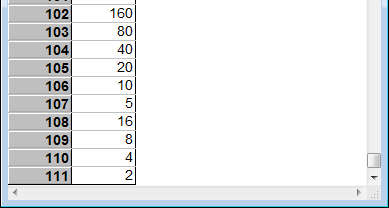The Linked list library is a library that comes with Analytica. To use it, you must add the library to your model by selecting Add Library... from the File menu. You can either Link or Embed the library, it shouldn't make much difference.

Linked lists can be of use in some algorithms that require a quick way to add items to a collection, when you have no way of determining in advance a ceiling on the maximum number of items, and when the algorithm is doing something more complex than simply extracting a subset of items in an array.

A linked list is a data structure containing an ordered list of items that enables quick prepending of new items to the front of the list, and reasonable quick "walking" of the items in sequential order, but slow random access to items. Do not confuse linked list with Analytica's native list data structure (See Alternatives below for more).

The special value Null is used as the zero-length linked list. Otherwise, a linked list is a reference to the first node of the linked list. Each item is stored in a node, which contains the item and a reference to the following node. The functions in the Linked list library isolate you from the specifics of internals of the data structure, but you will be exposed to the internals if you view a linked list in a result table. The linked list displays as «ref», and as you double click on each «ref», you can drill-down into the internals.

Each item in a linked list can be a scalar, array, list, or any other data structure.

## Using the Linked list library

Use a linked list within algorithms that need to collect an undetermined number of items, or when your algorithm needs to maintain a stack by pushing and popping context. With algorithms that collect items, after the algorithm has collected all the items, it is usually more convenient and more efficient to convert the linked list into an array (list) using LL_To_RArray or LL_To_Array, rather than to attempt to use the linked list directly as a collection of items. The linked list is efficient for collecting items, but the array is more efficient for accessing items, and the array is far more convenient since all other Analytica functions expect arrays and it can be viewed directly in a result table.

When collecting items, use LL_Push to push an item onto the front of an existing linked list. Start with Null as the empty list. Because LL_Push adds each new item to the front of the list, the linked list stores items in the reverse order that they are pushed.

When converting a linked list to an array, use LL_To_RArray to obtain an array with the items in the same order you "pushed" them, or LL_To_Array to obtain an array with the items in the reverse order that you pushed them.

The operations LL_Push, LL_First and LL_Remove_First are constant time operations. Hence, linked lists are useful when the core of your algorithm needs only these operations.

## Example

This example collects all numbers visited by the 3n + 1 algorithm when started from 27. The 3n + 1 algorithm starts at a whole number, then moves according to the rule: If n is even, halve it, if odd move to 3*n + 1. Terminate when you reach 1. It is a conjecture in number theory that the algorithm always terminates for any positive starting integer, but it remains an open problem to prove.

Var n := 27;
Var LL := null;
While (n > 1) do (
LL := LL_Push(LL, n);
n := If Mod(n, 2) Then 3*n + 1 Else n/2
);
LL_To_RArray(LL, "step")→⋮## Alternatives

A linked list is a data structure that is not the same as an Analytica list or array. You can "push" something on the front of a normal Analytica list using

Concat(x, L)

which in many cases is more direct, or on the rear of a list using Concat(L, x). However, when doing so, the elements of the list are copied with each push. When a large number of items are "pushed" onto a normal list in this fashion, your algorithm ends up with an $\displaystyle{ O(n^2) }$ time complexity. Using a linked list enables you to push n items in $\displaystyle{ O(n) }$ time.

If you are extracting a subset of items with a known property, Subset can be used, and will return a list directly, usually much faster than would be possible using a linked list to build up the list one element at a time.

Another alternative is to use an array. Start with an index, I that has more than the maximum number of items you would ever have in your collection, and then use slice assignment to add items to the array. This takes the form:

Var a := null;
Var n := 0;
a[I = (n := n + 1)] := 'a'; { Add 'a' as first element }
a[I = (n := n + 1)] := 'b'; { Add 'b' as second element }
...

Using an array with Slice assignment in this fashion is likely to be the most efficient method, but it does require you to have a bound on the maximum number of elements that can ever be in the collection, whereas when using a linked list, no such assumptions are necessary.

## Instructional value

Some programming languages have record or struct constructs that group data items into a single record. In Analytica, this is accomplished using an Index to define the members of the record, and references for non-scalar member items. One of the values of the linked list library is that it provides a simple example of using an index to define a record structure. The Linked_list index defines a record or struct for storing one node of the linked list. This record has two fields -- item and next.